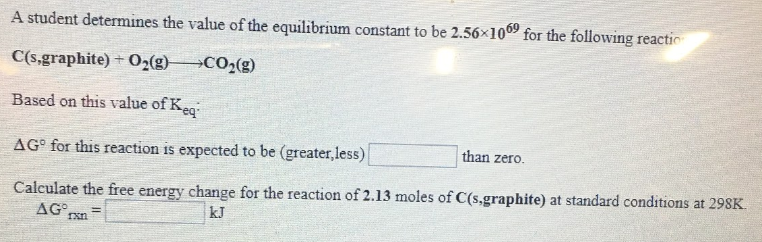# A student determines the value of the equilibrium constant to be 2.56x10^69 for the following reaction. C(s,graphite) + O2(g) → CO2(g) Based on this value of Keq: ΔG° for this reaction is expected to be (greater, less) ____ than zero. Calculate the free energy change for the reaction of 2.13 moles of C(s,graphite) at standard conditions at 298K. ΔG°rxn = ____ kJ.